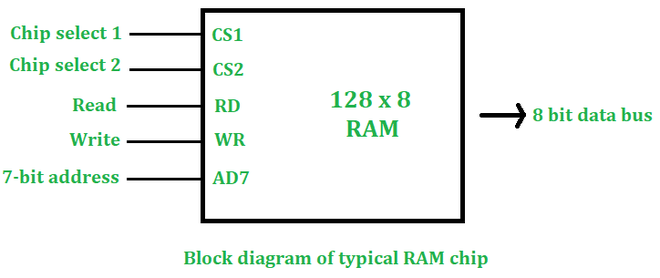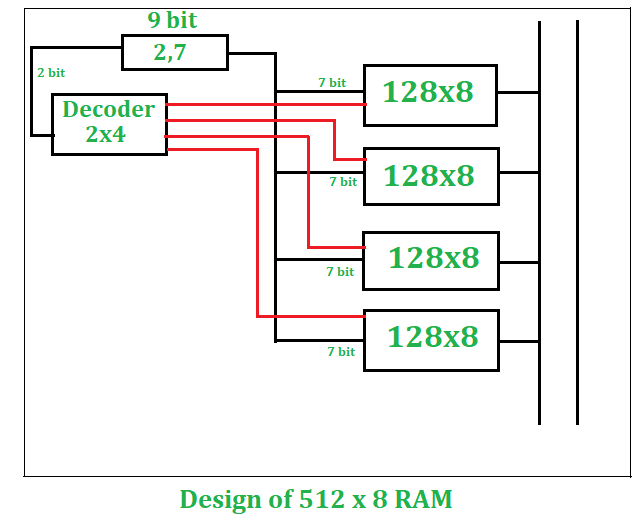GFG App
Open AppBrowser
Continue

# Design of 512×8 RAM using 128×8 RAM

If we see RAM physically then we notice that RAM is made up of a few chips. We can design the required RAM size using basic RAM chips. We can observe a basic RAM chip as follows:• CS1: For chip select 1, the value should be 1
• CS2: For chip select 2, the value should be 0.

Read and Write: Used for upcoming signals.

If we have n bit address and m bit words then our RAM size will be 2n x m.

Example:

```n=7 bit, m=8 bit
RAM size= 128 x 8 ```

Given:

```Basic RAM size = 128 x 8
Required RAM size  = 512 x 8```

To design a RAM size of 512×8 from 128×8, here are some calculations we need to do first:

1. Number of chips required:

```Number of chips required  = Desired RAM Size/ Basic RAM Size
=512x8/128x8
=4 chips```

```Required Size is 512 x 8
512 x 8= 29 x 8
Therefore, 9 bit address is required```

3. Decoder Size: If the number of words is increasing there will be a need for a decoder. In this case number of words is increasing (from 128 to 512).

```Size of decoder= number of times words increasing
128⇢512
words increased by 4 times
Decoder Size= 2x4```

Pictorial Description (Design of 512×8 RAM) is as follows:

From the above calculations, we see that we need four 128 x 8 RAM chips to design a 512 x 8 RAM size. If only the number of words is increasing then all chips will be arranged vertically. But, if the size of the data bit is also increasing, we have to horizontally arrange our new chip (For this case, we had to actually get the no. of column required using word size is desired / word size in the given chip. The no. of row required would be desired size/size in the given chip for example (512/128)). In this case, only the number of words is increasing (128-512) so all chips will be arranged vertically.

1. In a 512 x 8 RAM, there is a 9-bit address, we will divide this 9-bit address into 2 and 7-bit addresses.
2. The 2-bit address will be input as a 2 x 4 decoder, this decoder will have 4 output and the input will have 2 bits.
3. The 4 output of the decoder will enable every RAM of 128 x 8 individually.
4. Also, we can write 128 x 8 RAM chip as 27 x 8, every RAM chip will need a 7-bit address. We will connect the remaining 7-bit address line to every RAM.
5. This 7-bit address will select any word from the 128 words of that RAM, the selected word will be the output as the 8-bit word in the output bus.My Personal Notes arrow_drop_up